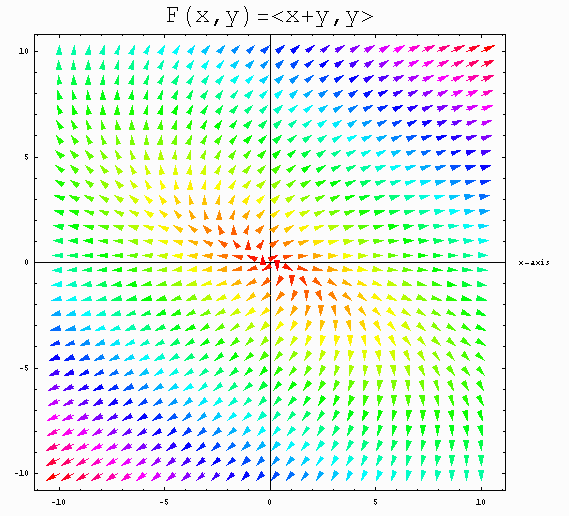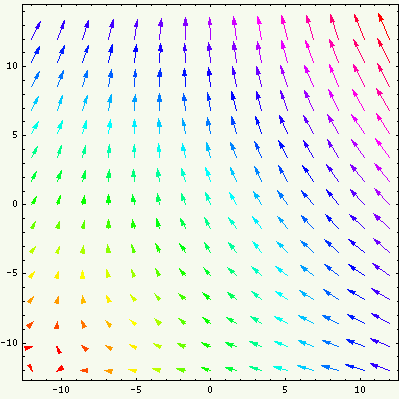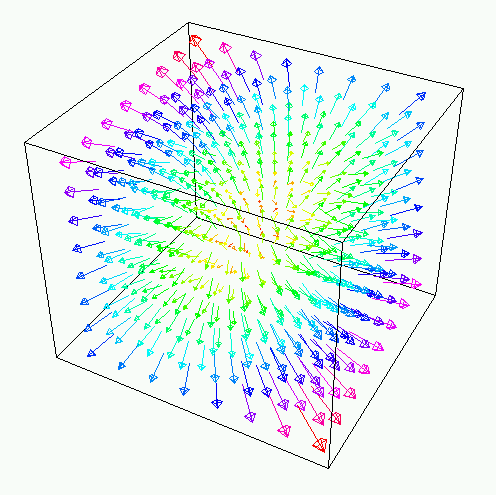```
```

# Plotting Vector Fields with MATHEMATICA

```
```

The MATHEMATICA command for sketching vector fields is   PlotVectorField . This command is in a special Graphics Package which is not automatically loaded by MATHEMATICA. So to use it you must first load the Graphics Package by entering the command

```        <<Graphics`PlotField`
```
(SPECIAL NOTE: in this command the word   PlotField   is immediately preceded and immediately followed by a left quotation mark ` (which may not show up very clearly in the HTML font used on this web page). There is no space before the first quotation mark.)   If you try to implement the   PlotVectorField   command before you have successfully loaded the Graphics Package with the << command, MATHEMATICA tends to get very confused. If this happens it is best to exit and start a new MATHEMATICA session.   Once the Graphics Package is loaded, you may use the command
PlotVectorField[ {P(x,y),Q(x,y)}, {x,xmin,xmax}, {y,ymin,ymax} ]
to sketch the 2-dimensional vector field   F(x,y) = P(x,y)i + Q(x,y)j   in the rectangle   [xmin,xmax] × [ymin,ymax] .

For example the output of the command

PlotVectorField[ {x+y,y}, {x,-10,10}, {y,-10,10} ]
looks like:To get a more controlled output we might add some modifiers such as

PlotVectorField[ {x+y,y}, {x,-10,10}, {y,-10,10}, ColorFunction->Hue, PlotPoints->30, Frame->True, PlotLabel->StyleForm["F(x,y)=<x+y,y>", FontSize->25], Axes->True, AxesLabel->{ "x-axis", " " } ]
obtaining:Note that the modifier ColorFunction->Hue colors vectors with equal magnitude with the same color.

Many of the modifiers used for the ContourPlot command will also work for PlotVectorField. Others may be found in MATHEMATICA's HELP utility by searching under Graphics`PlotField`. Here are some useful ones:

 AspectRatio -> NN set the aspect ratio to use in representing the viewing rectangle--the default is 1. Axes -> BB include or omit axes AxesLabel -> {"text","text"} label the axes ColorFunction -> Hue color the vectors as a function of magnitude PlotLabel -> "TEXT" create a label for the vector field plot PlotPoints -> NN number of points in each direction at which to draw vectors. The default is NN=15. ScaleFactor -> NN scales the magnitudes of displayed vectors--the default is NN=1. HeadWidth -> NN controls the size of the heads of the vectors--the default is NN=.5. HeadCenter -> NN controls the shape of the heads of the vectors--the default is NN=1. HeadLength -> NN allows more controls of the shape of the heads of the vectors. (Usually NN would be a very small positive number such as NN=.007)

In this table, NN denotes a numerical value, and the symbol BB takes one of the values True or False.

Here's an example showing how one might combine PlotVectorField with other graphics commands. This particular example displays a contour map for the function of two variables   f(x,y) = x^2 - y^2   with its gradient vector field superimposed. Of course the gradient vector field is orthogonal to the family contour curves for the function.

G1=ContourPlot[ x^2 - y^2, {x,-9,9}, {y,-9,9}, PlotPoints->35,
G2=PlotVectorField[ {2x,-2y}, {x,-8,8}, {y,-8,8},
ColorFunction->Hue, ScaleFactor->2];
Show[{G1,G2}, Background->RGBColor[.95, .95, .8] ]
obtaining:Special commands are available for plotting gradient vector fields and Hamiltonian vector fields:

PlotHamiltonianField[ {P(x,y),Q(x,y)}, {x,xmin,xmax}, {y,ymin,ymax} ]
The following example of this also illustrates how you might put graphics into motion:
Table[
PlotGradientField[ ((x-k)^2-(y-k)^2) *Cos[k/4]-2 (x-k)* (y-k)* Sin[k/4], {x,-12,12}, {y,-12,12},
ColorFunction->Hue,ScaleFactor->2,AspectRatio->1, Frame->True,Background->RGBColor[.94, .97, .92]]
,{k,-3.5Pi,3.5Pi,Pi/4}]
The output shows the gradient vector field for the function
f(x,y) = ((x-k)^2-(y-k)^2) Cos[k/4] - 2(x-k) (y-k) Sin[k/4]
as k ranges from -3.5 Pi to 3.5 Pi:It is also possible to have MATHEMTICA sketch 3-dimensional vector fields. For this you must first load the Graphics Package PlotField3D by entering

```        <<Graphics`PlotField3D`
```
Now use the command
PlotVectorField3D[ {P(x,y,z),Q(x,y,z),R(x,y,z)}, {x,xmin,xmax}, {y,ymin,ymax}, {z,zmin,zmax} ]
to sketch the 3-dimensional vector field   F(x,y,z) = P(x,y,z)i + Q(x,y,z)j + R(x,y,z)k   in the rectangular box   [xmin,xmax] × [ymin,ymax] × [zmin,zmax] . Here's a simple example
PlotVectorField3D[ {2x - z, z + y, y}, {x, -5, 5}, {y, -5, 5}, {z, -5, 5},
VectorHeads -> True, ScaleFactor -> 2.5, ColorFunction -> Hue, ColorFunction -> Hue, PlotPoints -> 8]
whose output looks like```
```

URL: http://math.ou.edu/~amiller/math/vfield.htm

November, 1999